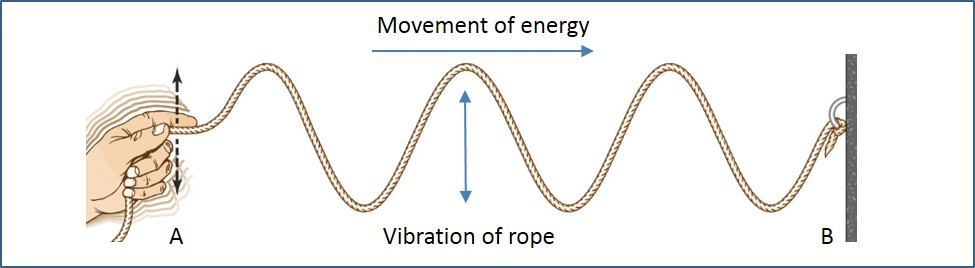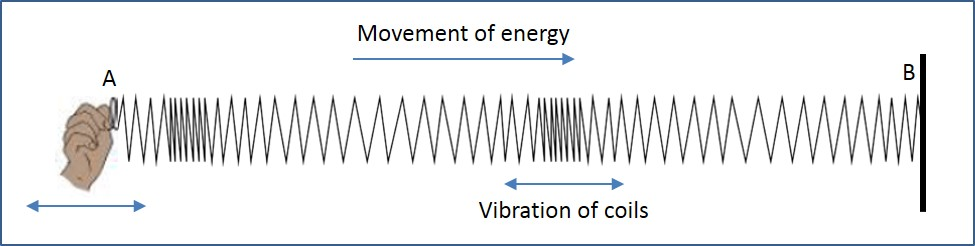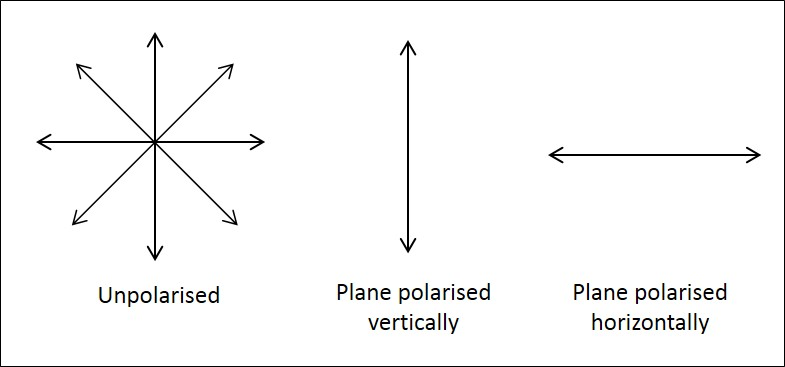# Longitudinal & Transverse Waves

## Introduction

Wave motion is a means of moving energy from place to place. For example: the electromagnetic waves from the sun carry the energy to the Earth for the survival of living things. The energy from sound reaches our eardrums to vibrate. Waves that move energy from place to place without the transfer of matter are called progressive waves. Vibrating objects act as a source of waves. For example, a vibrating tuning fork sets the air close to it into oscillation, and a sound wave spreads out from the fork. In this article, two main groups of waves are explained.

A. Transverse Waves

A transverse wave is one in which vibrations of the particles in the wave are at right angles to the direction in which the energy of the wave is travelling.

Figure 1 shows a transverse wave moving along a rope. The particles of the rope vibrate up and down, while the energy travels at right angles to this, from A to B. There is no transfer of matter from A to B. Examples of transverse waves include surface water waves and secondary seismic waves (S-waves).Figure 1: A Transverse Wave

B. Longitudinal Waves

A longitudinal wave is one in which the direction of the vibrations of the particles in the wave is along the direction in which the energy of the wave is travelling. Figure 2 shows a longitudinal wave moving along a stretched spring. The coils of the spring vibrate along the length of the spring, while energy travels along the same line, from A to B. Note that the spring itself does not move from A to B.

Examples of longitudinal waves include sound waves and primary seismic waves (P-waves). In transverse waves, the ‘particles’ of the wave oscillate in a line at right angles to the direction of travel. Light waves are an example of longitudinal waves.Figure 2: Longitudinal Wave

C. Polarisation

Polarisation is the production of waves oscillating in one plane with respect to the line of oscillation from a source of randomly oscillating waves. The different types of oscillation of waves are illustrated in Figure 3.Figure 3: Oscillation of Waves

Polarisation is done by passing the wave through a grid so that only the waves that can fit through the slits continue through. Polarising of a light wave is illustrated in the following figure.Figure 4: Polarisation of Light Waves

Let us understand the process of polarisation of a light wave. Polaroid is a material made of plastic. Long molecules of quinine iodo sulphate are lined up so that the light wave oscillating in one particular direction can pass through. When an unpolarized beam of light is directed at the polaroid, as shown in Figure 4, a beam of vertically polarized light waves is transmitted. Two pieces of polaroid that have molecules aligned at 90° to each other will not allow any light through.

D. Summary

• Waves, which move energy from place to place without the transfer of matter, are called progressive waves.
• Two main groups of waves are longitudinal waves and transverse waves.
• A transverse wave is one in which vibrations of the particles in the wave are at right angles to the direction in which the energy of the wave is travelling.
• A longitudinal wave is one in which the direction of the vibrations of the particles in the wave is along the direction in which the energy of the wave is travelling.
• Polarisation is the production of waves oscillating in one plane with respect to the line of oscillation from a source of randomly oscillating waves.